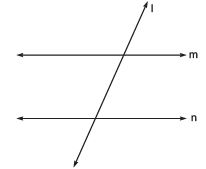Courses

# Ex 5.2 NCERT Solutions - Introduction to Euclid’s Geometry Class 9 Notes | EduRev

## Class 9 Mathematics by Full Circle

Created by: Full Circle

## Class 9 : Ex 5.2 NCERT Solutions - Introduction to Euclid’s Geometry Class 9 Notes | EduRev

The document Ex 5.2 NCERT Solutions - Introduction to Euclid’s Geometry Class 9 Notes | EduRev is a part of the Class 9 Course Class 9 Mathematics by Full Circle.
All you need of Class 9 at this link: Class 9

Question 1. How would you rewrite Euclid’s fifth postulate so that it would be easier to understand?
Solution: We can have: “Two distinct intersecting lines cannot be parallel to the same line.”

Question 2. Does Euclid’s fifth postulate imply the existence of parallel lines? Explain.
Solution: Yes, If a straight line ‘l’ falls on two lines ‘m’ and ‘n’ such that sum of the interior angles on one side of l is two right angles, then by Euclid’s fifth postulate, the lines will not meet on this side of l. Also we know that the sum of the interior angles on the other side of the line l will be two right angles too. Thus, they will not meet on the other side also.∴ The lines ‘m’ and ‘n’ never meet, i.e., they are parallel.

132 docs

,

,

,

,

,

,

,

,

,

,

,

,

,

,

,

,

,

,

,

,

,

;